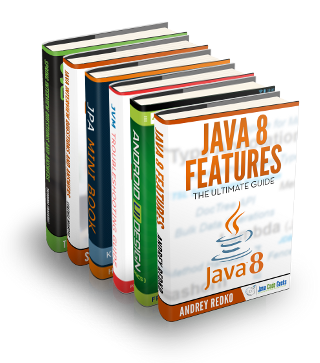Home » Java » Core Java » Java 8 Friday Goodies: Easy-as-Pie Local CachingLukas is a Java and SQL enthusiast developer. He created the Data Geekery GmbH. He is the creator of jOOQ, a comprehensive SQL library for Java, and he is blogging mostly about these three topics: Java, SQL and jOOQ.

# Java 8 Friday Goodies: Easy-as-Pie Local Caching

At Data Geekery, we love Java. And as we’re really into jOOQ’s fluent API and query DSL, we’re absolutely thrilled about what Java 8 will bring to our ecosystem. We have blogged a couple of times about some nice Java 8 goodies, and now we feel it’s time to start a new blog series, the…

## Java 8 Friday

Every Friday, we’re showing you a couple of nice new tutorial-style Java 8 features, which take advantage of lambda expressions, extension methods, and other great stuff. You’ll find the source code on GitHub.

## Java 8 Goodie: Easy-as-Pie Local Caching

Now get ready for one of the most awesome revelations in this series so far. We’ll show you an easy-as-pie way to implement a local cache using the good old `ConcurrentHashMap` and lambda expressions. Because, `Map` now has a new way of a atomically calculating a new value in case a key is absent. Perfect for caches. Let’s delve into code:

```public static void main(String[] args) {
for (int i = 0; i < 10; i++)
System.out.println(
"f(" + i + ") = " + fibonacci(i));
}

static int fibonacci(int i) {
if (i == 0)
return i;

if (i == 1)
return 1;

System.out.println("Calculating f(" + i + ")");
return fibonacci(i - 2) + fibonacci(i - 1);
}```

Yes. That’s the naive way of doing things. Even for small numbers like `fibonacci(5)`, the above algorithm will print out a huge amount of lines, as we’re repeating the same calculations exponentially:

```Calculating f(6)
Calculating f(4)
Calculating f(2)
Calculating f(3)
Calculating f(2)
Calculating f(5)
Calculating f(3)
Calculating f(2)
Calculating f(4)
Calculating f(2)
Calculating f(3)
Calculating f(2)
f(6) = 8```

What we want to do is build a cache of previously calculated fibonacci numbers. The most straightforward technique is to memoize all values in a cache. Here’s how we build a cache:

```static Map<Integer, Integer> cache
= new ConcurrentHashMap<>();```

Done! As mentioned before, we’re using the newly added `Map.computeIfAbsent()` method to calculate a new value from a `source` function only if we don’t already have a value for a given key. Caching! And since this method is guaranteed to execute atomically, and since we’re using a `ConcurrentHashMap`, this cache is even thread-safe without resorting to manually applying `synchronized` anywhere. And it can be reused for stuff other than calculating fibonacci numbers. But let’s first apply this cache to our `fibonacci()` function.

```static int fibonacci(int i) {
if (i == 0)
return i;

if (i == 1)
return 1;

return cache.computeIfAbsent(i, (key) ->
fibonacci(i - 2)
+ fibonacci(i - 1));
}```

That’s it. It can’t get any simpler than this! Want proof? We’ll log a message on the console every time we actually evaluate a new value:

```static int fibonacci(int i) {
if (i == 0)
return i;

if (i == 1)
return 1;

return cache.computeIfAbsent(i, (key) -> {
System.out.println(
"Slow calculation of " + key);

return fibonacci(i - 2) + fibonacci(i - 1);
});
}```

The above program will print

```f(0) = 0
f(1) = 1
Slow calculation of 2
f(2) = 1
Slow calculation of 3
f(3) = 2
Slow calculation of 4
f(4) = 3
Slow calculation of 5
f(5) = 5
Slow calculation of 6
f(6) = 8
Slow calculation of 7
f(7) = 13
Slow calculation of 8
f(8) = 21
Slow calculation of 9
f(9) = 34```

## How would we have done it in Java 7?

Good question. With lots of code. We’d probably write something like this using double-checked locking:

```static int fibonacciJava7(int i) {
if (i == 0)
return i;

if (i == 1)
return 1;

Integer result = cache.get(i);
if (result == null) {
synchronized (cache) {
result = cache.get(i);

if (result == null) {
System.out.println(
"Slow calculation of " + i);

result = fibonacci(i - 2)
+ fibonacci(i - 1);
cache.put(i, result);
}
}
}

return result;
}```

Convinced?

Note, that your actual solution would probably make use of Guava Caches.

## Conclusion

Lambdas are only one part of Java 8. An important part, but let’s not forget all the new features that were added to the libraries and that can be leveraged with lambdas now!

This is really exciting and…

We can greatly improve our code bases without resorting to new libraries. All of the above can be run with the JDK’s libraries only.

Next week in this blog series, we’re going to look at how Java 8 will improve on the existing and new concurrency APIs, so stay tuned!

## More on Java 8

In the mean time, have a look at Eugen Paraschiv’s awesome Java 8 resources page(0 rating, 0 votes)
You need to be a registered member to rate this.
Start the discussion Views Tweet it!
Do you want to know how to develop your skillset to become a Java Rockstar?
Subscribe to our newsletter to start Rocking right now!
To get you started we give you our best selling eBooks for FREE!
1. JPA Mini Book
2. JVM Troubleshooting Guide
3. JUnit Tutorial for Unit Testing
4. Java Annotations Tutorial
5. Java Interview Questions
6. Spring Interview Questions
7. Android UI Design
and many more ....